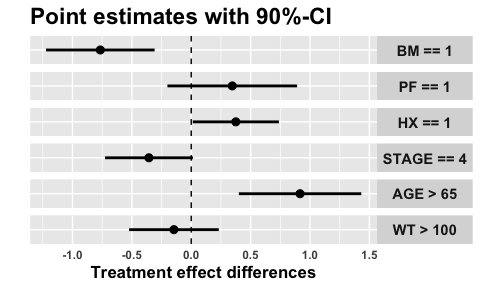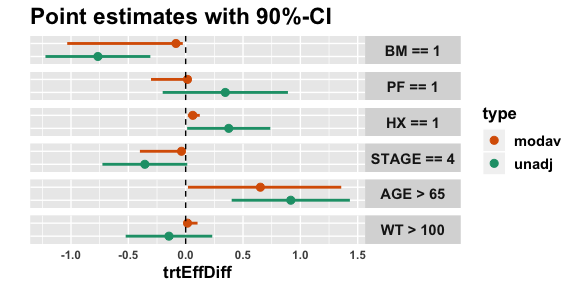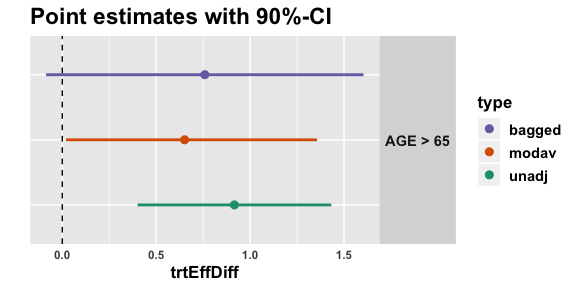The subtee plot function

2020-09-02

In this vignette we showcase the various plots can be made with the package.

Analyzing the data

We first start producing the treatment effect estimates for all subgroups, using the unadj, modav and bagged functions.

library(ggplot2)
library(subtee)
################################################################################
# We use the dataset from Rosenkranz (2016) https://onlinelibrary.wiley.com/doi/abs/10.1002/bimj.201500147
# to illustrate the methods proposed in this work.
# The data comes from a clinical trial of an prostate cancer
# treatment
# Data is loaded from  Royston, Patrick, and Willi Sauerbrei.
# Multivariable model-building: a pragmatic approach to
# regression anaylsis based on fractional polynomials for
# modelling continuous variables. Vol. 777. John Wiley & Sons, 2008.
# https://www.imbi.uni-freiburg.de/Royston-Sauerbrei-book
prca = get_prca_data()

## first create candidate subgroups
cand.groups <- subtee::subbuild(prca, dupl.rm = TRUE,
BM == 1, PF == 1, HX == 1,
STAGE == 4, AGE > 65, WT > 100)
fitdat <- cbind(prca, cand.groups)
subgr.names = names(cand.groups)
prog = as.formula(paste(" ~ ", paste0("", names(cand.groups),"", collapse = " + ")))

data = fitdat, covars = prog,
event = "CENS", fitfunc = "coxph")
### ModelAveraging estimates
res_modav = modav(resp = "SURVTIME", trt = "RX", subgr = subgr.names,
data = fitdat, covars = prog,
event = "CENS", fitfunc = "coxph")
### Bagged estimates
set.seed(321231) # set seed for reproducible results in the bootstrap samples
res_bagged = bagged(resp = "SURVTIME", trt = "RX", subgr = subgr.names,
data = fitdat, covars = prog,
event = "CENS", fitfunc = "coxph",
select.by = "BIC", B = 200) #B = 2000)

Plotting treatment effect estimates in subgroups

The objects resulting from calling unadj, modav and bagged are subtee objects that contain the results in a format that can be used to produce plots. For example, the following produces a forest plot showing treatment effect estimates for the subgroups and their complements:

ggplot(aes(y = Subset, x = trtEff, xmin = LB, xmax = UB, colour = Subset),
geom_point(size = 2) +
geom_errorbarh(size = 1, show.legend = FALSE, height = 0) +
facet_grid(Group ~ .)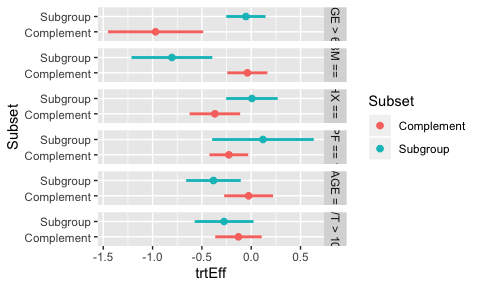Using the generic plot function provided in the package

The default option for the generic plot function in the package for subtee objects shows the treatment effect in subgroups along with their confidence intervals.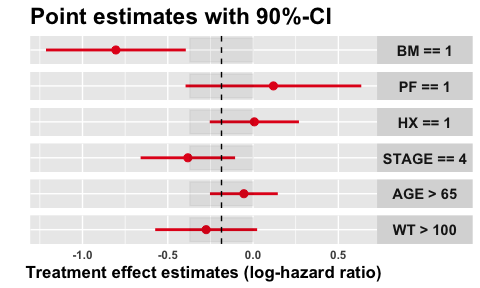Note that only the treatment effect estimates in subgroups are displayed. Setting the option show.compl = TRUE displays the treatment effect estimates in both subgroups and complements.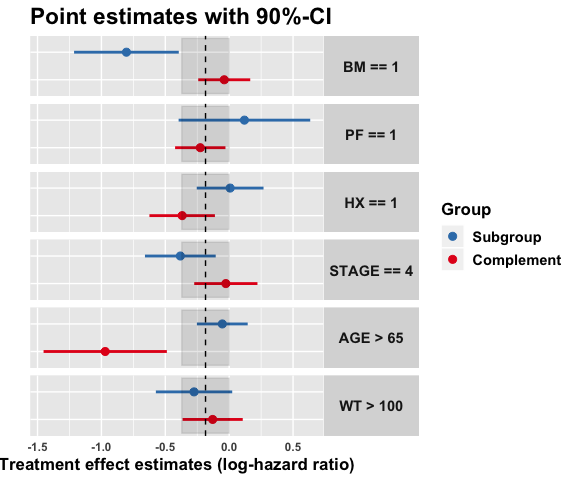When using the plot function to subtee objects with unadjusted or model averaging estimates, the same layout is used. However, when the a subtee object generated with the bagged funciton is provided. it will only show the selected subgroup.

plot(res_bagged, show.compl = TRUE)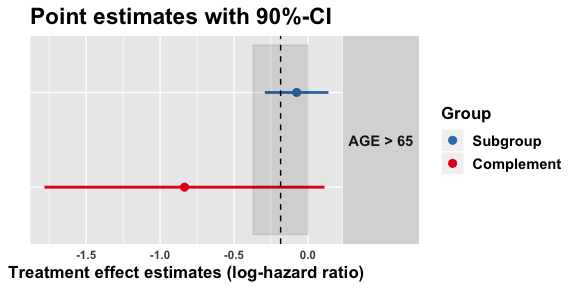Comparing different estimation techniques

When more than one object is provided, the plot shows the comparison between different estimation techniques.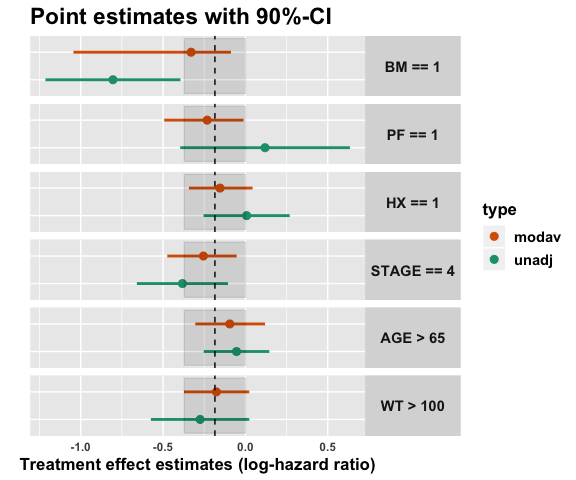In this case it is again possible to set show.compl = TRUE.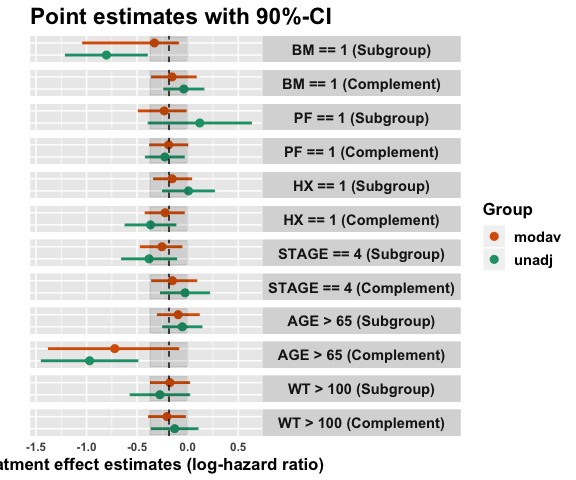And if bagged estimates are provided, it will only show the selected subgroup.

plot(res_unadj, res_modav, res_bagged, show.compl = TRUE)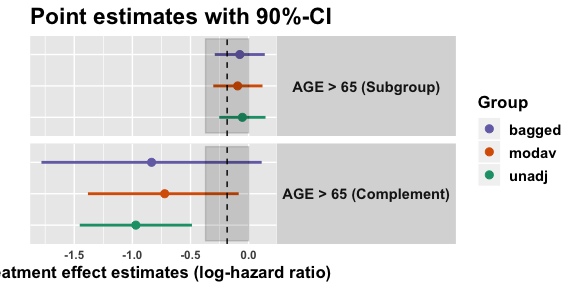Plotting treatment effect difference between subgroup and complement

The plot function has also the option to show the treatment effect difference between subgroup and complement setting type = "trtEffDiff".